Want to share your content on R-bloggers? click here if you have a blog, or here if you don't.

In ggplot2, aesthetics and their `scale_*()` functions change both the plot appearance and the plot legend appearance simultaneously. The `override.aes` argument in `guide_legend()` allows the user to change only the legend appearance without affecting the rest of the plot. This is useful for making the legend more readable or for creating certain types of combined legends.

In this post I’ll first introduce `override.aes` with a basic example and then go through three additional plotting scenarios to how other instances where `override.aes` comes in handy.

# R packages

The only package I’ll use in this post is ggplot2 for plotting.

`library(ggplot2) # v. 3.3.2`

# Introducing override.aes

A basic reason to change the legend appearance without changing the plot is to make the legend more readable.

For example, I’ll start with a scatterplot using the `diamonds` dataset. This is a large dataset, so after mapping `color` to the `cut` variable I set `alpha` to increase the transparency and `size` to reduce the size of points in the plot.

You can see using `alpha` and `size` changes the way the points are shown in both the plot and the legend.

```ggplot(data = diamonds, aes(x = carat, y = price, color = cut) ) +
geom_point(alpha = .25, size = 1)```Making the points small and transparent may be desirable when plotting many points, but it also makes the legend more difficult to read. This is a case where I’d want to make the legend more readable by increasing the point size and/or reducing the point transparency.

One way to do this is by adding a `guides()` layer. The `guides()` function uses scale name-guide pairs. I am going to change the legend for the `color` scale, so I’ll use `color = guide_legend()` as the scale name-guide pair.

`override.aes` is an argument within `guide_legend()`, so if you’re looking for more background you can start at `?guide_legend`. The `override.aes` argument takes a list of aesthetic parameters that will override the default legend appearance.

To increase the `size` of the points in the `color` legend of my plot, the layer I’ll add will look like:

`guides(color = guide_legend(override.aes = list(size = 3) ) )`

Adding this layer to the initial plot, you can see how the points in the legend get larger while the points in the plot remain unchanged.

```ggplot(data = diamonds, aes(x = carat, y = price, color = cut) ) +
geom_point(alpha = .25, size = 1) +
guides(color = guide_legend(override.aes = list(size = 3) ) )```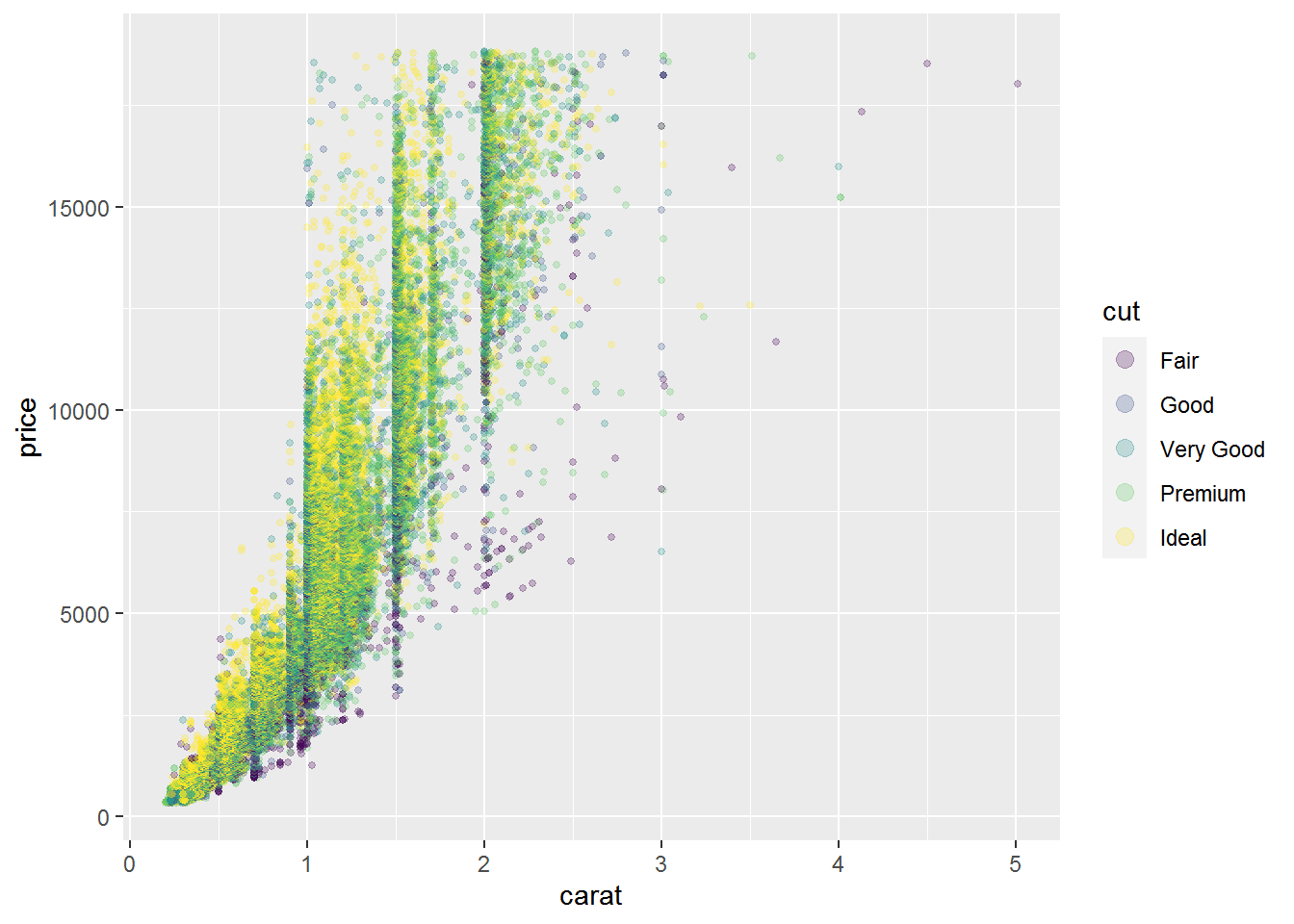## Using the guide argument in scale_*()

If I am going to change my default colors with a `scale_color_*()` function in addition to overriding the legend appearance, I can use the `guide` argument there instead of adding a separate `guides()` layer. The `guide` argument is part of all scale functions.

For example, say I am already using `scale_color_viridis_d()` to change the default color palette of the whole plot (i.e., plot and legend). I can use the same `guide_legend()` code from above for the `guide` argument to change the size of the points in the legend.

```ggplot(data = diamonds, aes(x = carat, y = price, color = cut) ) +
geom_point(alpha = .25, size = 1) +
scale_color_viridis_d(option = "magma",
guide = guide_legend(override.aes = list(size = 3) ) )```## Changing multiple aesthetic parameters

You can control multiple aesthetic parameters at once by adding them to the list passed to `override.aes`. If I want to increase the point size as well as remove the point transparency in the legend, I can change both `size` and `alpha`.

```ggplot(data = diamonds, aes(x = carat, y = price, color = cut) ) +
geom_point(alpha = .25, size = 1) +
scale_color_viridis_d(option = "magma",
guide = guide_legend(override.aes = list(size = 3,
alpha = 1) ) )```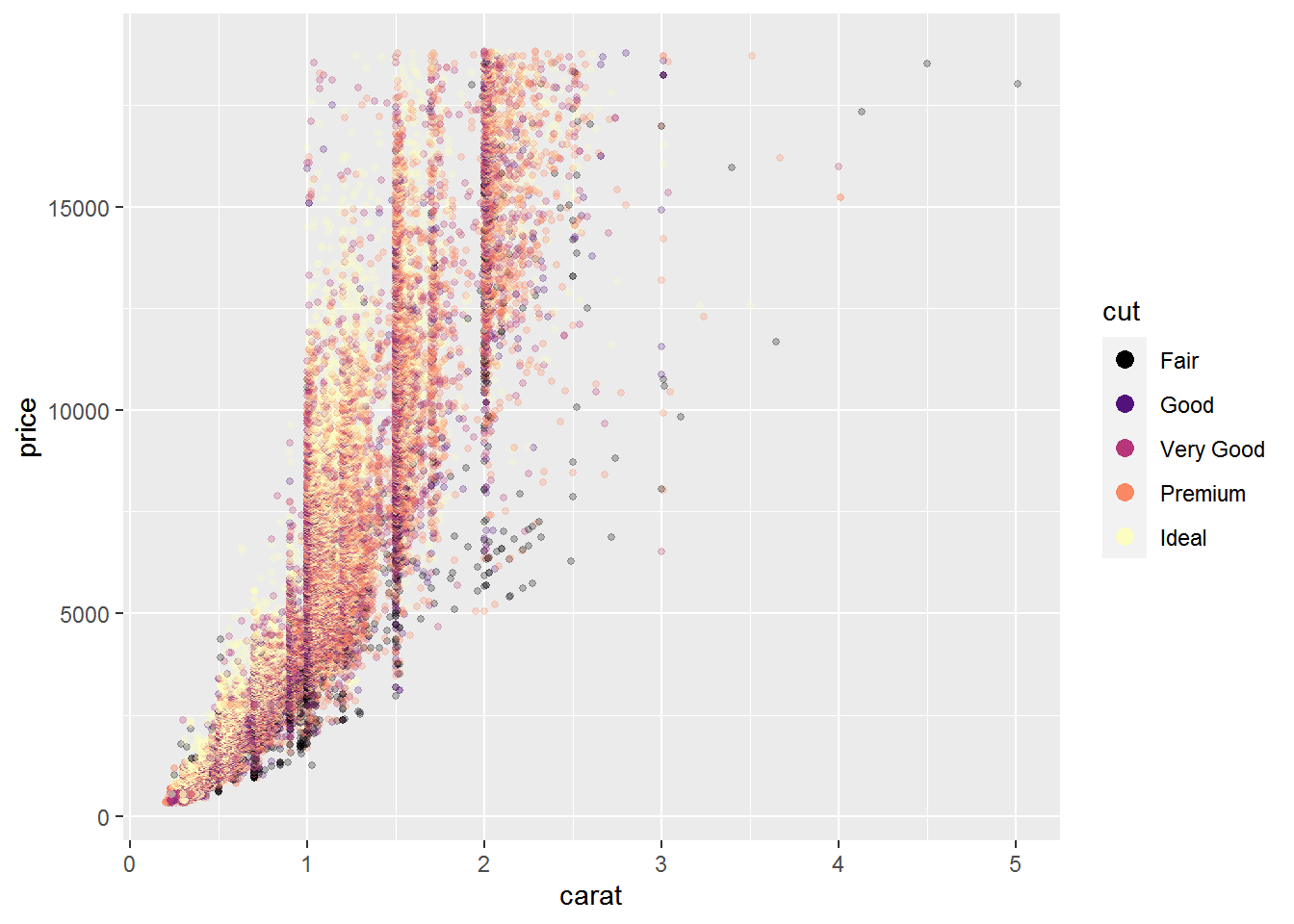# Suppress aesthetics from part of the legend

Removing aesthetic for only some parts of the legend is another use for `override.aes`. For example, this can be useful when different layers are based on a different number of levels for the same grouping factor.

The following example is based on this Stack Overflow question. The `points` data has information from all three groups of the `id` variable but the rectangle, based on the `box` dataset, is for only a single group.

```points = structure(list(x = c(5L, 10L, 7L, 9L, 86L, 46L, 22L, 94L, 21L,
6L, 24L, 3L), y = c(51L, 54L, 50L, 60L, 97L, 74L, 59L, 68L, 45L,
56L, 25L, 70L), id = c("a", "a", "a", "a", "b", "b", "b", "b",
"c", "c", "c", "c")), row.names = c(NA, -12L), class = "data.frame")

#    x  y id
# 1  5 51  a
# 2 10 54  a
# 3  7 50  a
# 4  9 60  a
# 5 86 97  b
# 6 46 74  b
box = data.frame(left = 1, right = 10, bottom = 50, top = 60, id = "a")
box
#   left right bottom top id
# 1    1    10     50  60  a```

Here’s what the initial plot looks like, mapping `color` to the `id` variable. Note that the colored outlines, representing the rectangle layer, are present for every group in the legend even though there is a rectangle for only one of the groups present in the plot.

```ggplot(data = points, aes(color = id) ) +
geom_point(aes(x = x, y = y), size = 4) +
geom_rect(data = box, aes(xmin = left,
xmax = right,
ymin = 50,
ymax = top),
fill = NA, size = 1)```In this case, I want to remove the outlines for the second and third legend key boxes so the legend matches what is in the plot. The legend outlines are based on the `linetype` aesthetic. Suppressing these lines can be done with `override.aes`, setting the line types to `0` in order to remove them.

Note that I have to list the line type for every group, not just the groups I want to remove. I keep the line for the first group solid via `1`.

```ggplot(data = points, aes(color = id) ) +
geom_point(aes(x = x, y = y), size = 4) +
geom_rect(data = box, aes(xmin = left,
xmax = right,
ymin = 50,
ymax = top),
fill = NA, size = 1) +
guides(color = guide_legend(override.aes = list(linetype = c(1, 0, 0) ) ) )```# Combining legends from two layers

In the next example I’ll show how `override.aes` can be useful when creating a legend based on multiple layers and want distinct symbols in each legend key box.

There are situations where we want to add a legend to identify different elements of the plot, such as indicating the plotted line is a fitted line or that points are means. This can be done by mapping aesthetics to constants to make a manual legend and then manipulating the symbols shown in the legend via `override.aes`. I wrote about making manual legends in an earlier blog post.

The plot below shows observed values and a fitted line per group based on `color`.

```ggplot(data = mtcars, aes(x = mpg, y = wt, color = factor(am) ) ) +
geom_point(size = 3) +
geom_smooth(method = "lm", se = FALSE)
# `geom_smooth()` using formula 'y ~ x'```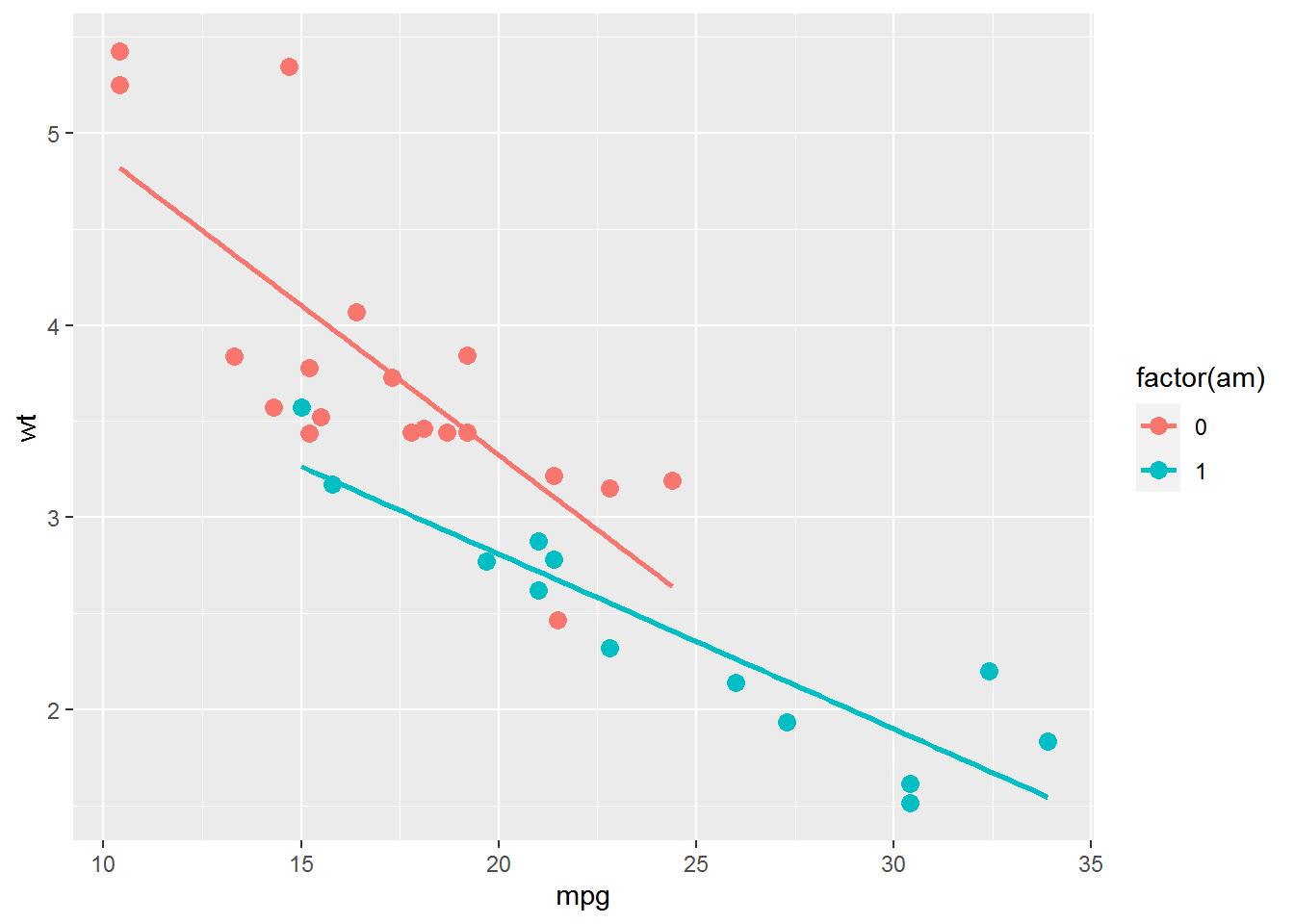I’m going to leave the `color` legend alone but I want to add a second legend to indicate that the points are observed values and the lines are fitted lines. I’ll use the `alpha` aesthetic for this. Using an aesthetic that you haven’t used already and affects both layers is a trick that often comes in handy when adding an extra legend like I’m doing here.

I don’t actually want `alpha` to affect the plot appearance, so I also add `scale_alpha_manual()` to make sure both layers stay opaque by setting the `values` for both groups to 1. I also remove the legend name and set the order of the `breaks` so the `Observed` group is listed first in the new legend.

```ggplot(data = mtcars, aes(x = mpg, y = wt, color = factor(am) ) ) +
geom_point(aes(alpha = "Observed"), size = 3) +
geom_smooth(method = "lm", se = FALSE, aes(alpha = "Fitted") ) +
scale_alpha_manual(name = NULL,
values = c(1, 1),
breaks = c("Observed", "Fitted") )```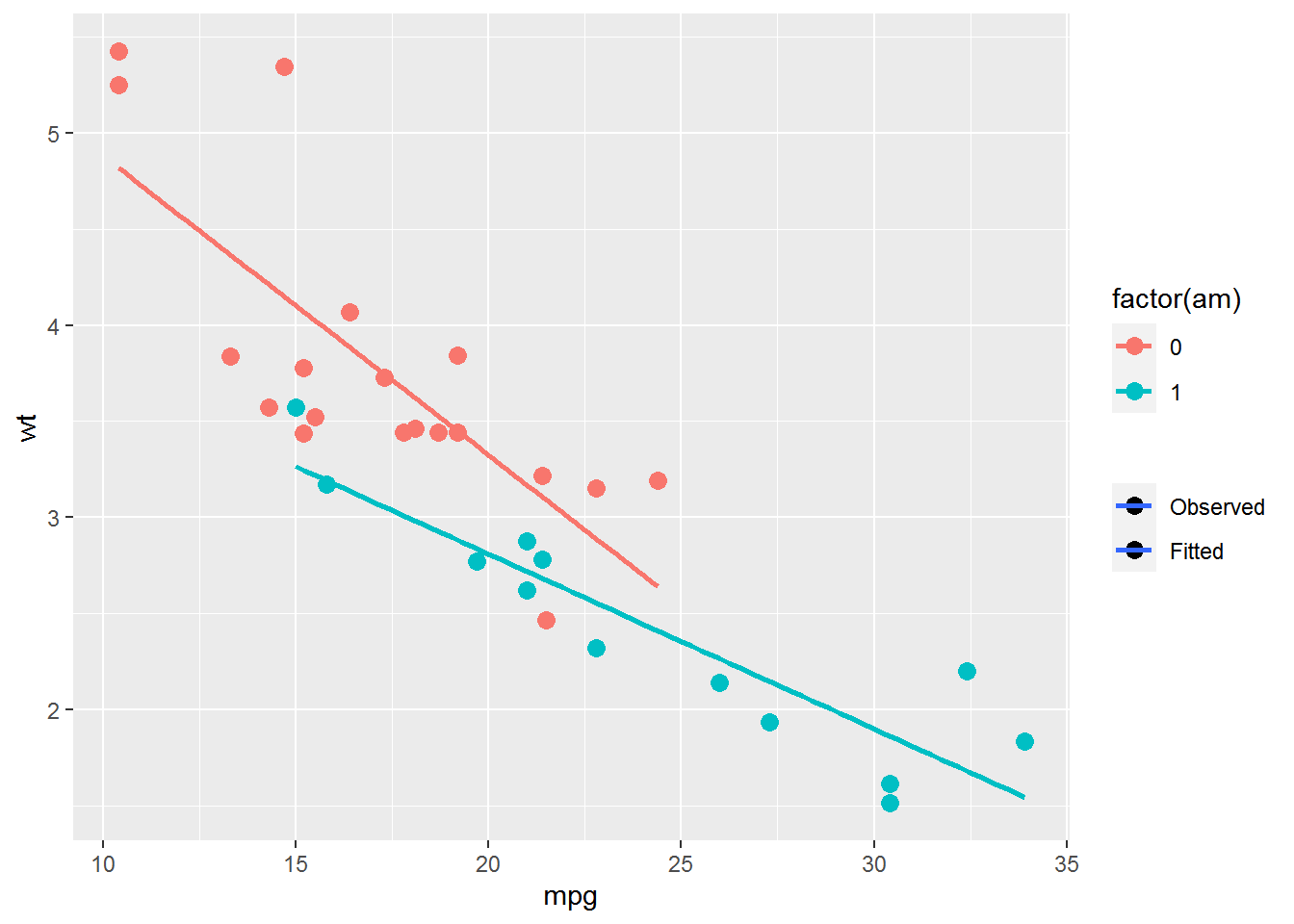Now I have a new legend to work with. However, the legend has both the point and the line symbol in all legend key boxes. I need to override the current legend so the `Observed` legend key box contains only a point symbol and the `Fitted` legend key box has only a line symbol. This is where `override.aes` comes in.

Here’s what I’ll do: I’ll change the `linetype` to `0` for the first key box but leave it as `1` for the second. I’ll use shape `16` (a solid circle) as the `shape` for the first key box but remove the point all together in the second key box with `NA`. I’m also going to make sure all elements are black via `color`. (If you need to know codes for shapes and line types see here.)

I use `linetype`, `shape`, and `color` in the `override.aes` list within `scale_alpha_manual()`.

```ggplot(data = mtcars, aes(x = mpg, y = wt, color = factor(am) ) ) +
geom_point(aes(alpha = "Observed"), size = 3) +
geom_smooth(method = "lm", se = FALSE, aes(alpha = "Fitted") ) +
scale_alpha_manual(name = NULL,
values = c(1, 1),
breaks = c("Observed", "Fitted"),
guide = guide_legend(override.aes = list(linetype = c(0, 1),
shape = c(16, NA),
color = "black") ) )```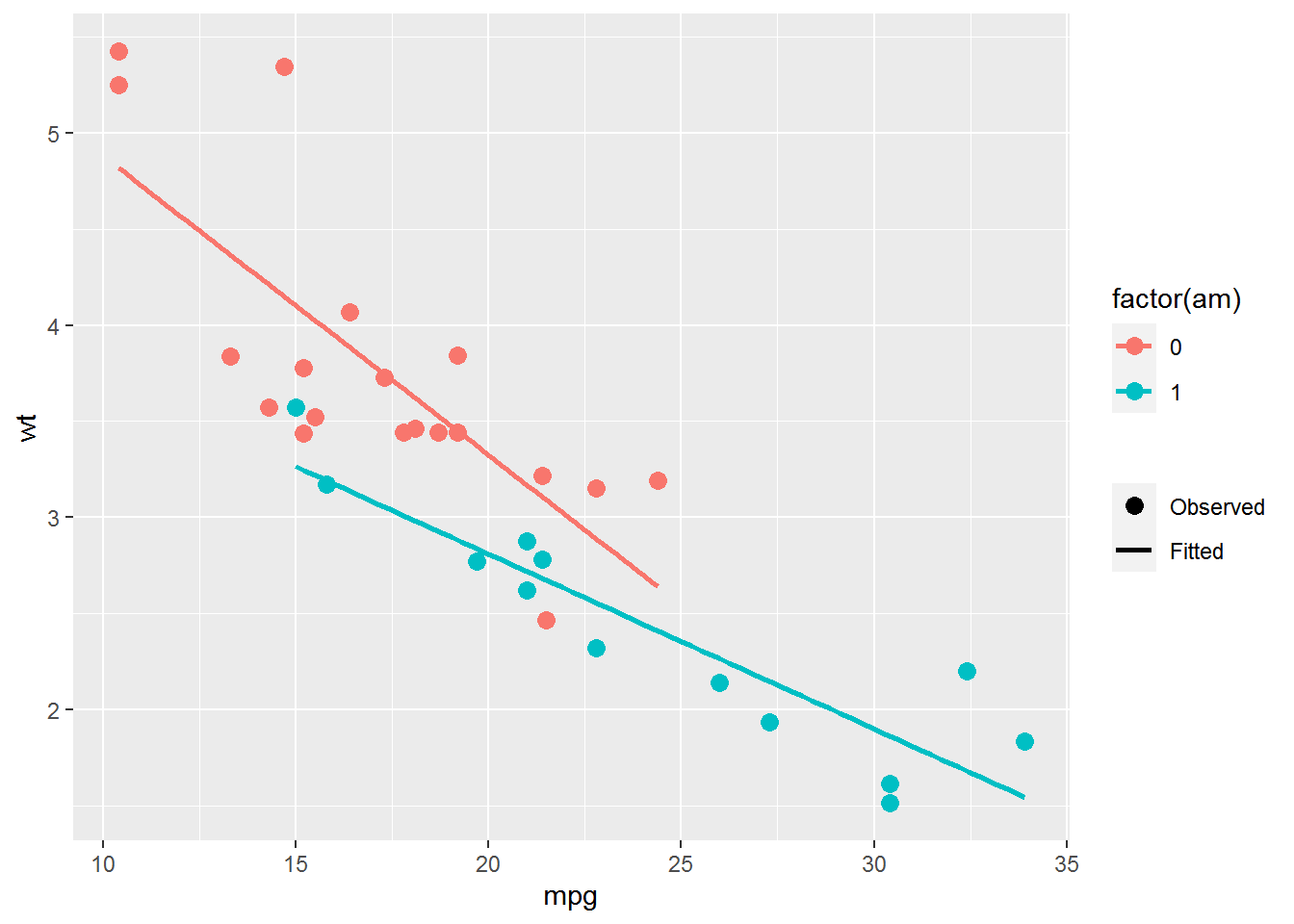# Controlling the appearance of multiple legends

The final example for `override.aes` may seem a little esoteric, but it has come up for me in the past. Say I want to make a scatterplot with the `fill` and `shape` aesthetics mapped to two different factors. I use `fill` instead of `color` so the points have an outline. Having an outline around the points can matter if, e.g., you have a white plot background and wanted the points to be black and white as in this question.

This is the dataset I’ll use in this plot example, where the two factors are named `g1` and `g2`.

```dat = structure(list(g1 = structure(c(1L, 2L, 1L, 2L, 1L, 2L, 1L, 2L,
1L, 2L, 1L, 2L, 1L, 2L, 1L, 2L), class = "factor", .Label = c("High",
"Low")), g2 = structure(c(1L, 1L, 2L, 2L, 1L, 1L, 2L, 2L, 1L,
1L, 2L, 2L, 1L, 1L, 2L, 2L), class = "factor", .Label = c("Control",
"Treatment")), x = c(0.42, 0.39, 0.56, 0.59, 0.17, 0.95, 0.85,
0.25, 0.31, 0.75, 0.58, 0.9, 0.6, 0.86, 0.61, 0.61), y = c(-1.4,
3.6, 1.1, -0.1, 0.5, 0, -1.8, 0.8, -1.1, -0.6, 0.2, 0.3, 1.1,
1.6, 0.9, -0.6)), class = "data.frame", row.names = c(NA, -16L
))

#     g1        g2    x    y
# 1 High   Control 0.42 -1.4
# 2  Low   Control 0.39  3.6
# 3 High Treatment 0.56  1.1
# 4  Low Treatment 0.59 -0.1
# 5 High   Control 0.17  0.5
# 6  Low   Control 0.95  0.0```

I set the colors for the `fill` in `scale_fill_manual()` and choose fillable shapes in `scale_shape_manual()`. Fillable shapes are shapes 21 through 25.

```ggplot(data = dat, aes(x = x, y = y, fill = g1, shape = g2) ) +
geom_point(size = 5) +
scale_fill_manual(values = c("#002F70", "#EDB4B5") ) +
scale_shape_manual(values = c(21, 24) )```The plots itself shows the fill colors and shapes, but you can some issues in the legends. The fill colors don’t show up in the `g1` legend at all. This is because the default shape in the legend isn’t a fillable shape. In addition, the `g2` legend shows unfilled points and I think it would look better if the points were filled.

I can address both these issues via `override.aes`. I’ll change the point shape in the `fill` legend to shape 21 and the fill color in the `shape` legend to black within a `guides()` layer. This code gives you a chance to see how you can use use multiple scale name-guide pairs within the same `guides()` layer.

```ggplot(data = dat, aes(x = x, y = y, fill = g1, shape = g2) ) +
geom_point(size = 5) +
scale_fill_manual(values = c("#002F70", "#EDB4B5") ) +
scale_shape_manual(values = c(21, 24) ) +
guides(fill = guide_legend(override.aes = list(shape = 21) ),
shape = guide_legend(override.aes = list(fill = "black") ) )```While I’m sure you can come up with additional scenarios, that should give you a taste for when overriding the aesthetics in the legend is useful. ????

Here’s the code without all the discussion. Copy and paste the code below or you can download an R script of uncommented code from here.

```library(ggplot2) # v. 3.3.2

ggplot(data = diamonds, aes(x = carat, y = price, color = cut) ) +
geom_point(alpha = .25, size = 1)

ggplot(data = diamonds, aes(x = carat, y = price, color = cut) ) +
geom_point(alpha = .25, size = 1) +
guides(color = guide_legend(override.aes = list(size = 3) ) )

ggplot(data = diamonds, aes(x = carat, y = price, color = cut) ) +
geom_point(alpha = .25, size = 1) +
scale_color_viridis_d(option = "magma",
guide = guide_legend(override.aes = list(size = 3) ) )

ggplot(data = diamonds, aes(x = carat, y = price, color = cut) ) +
geom_point(alpha = .25, size = 1) +
scale_color_viridis_d(option = "magma",
guide = guide_legend(override.aes = list(size = 3,
alpha = 1) ) )

points = structure(list(x = c(5L, 10L, 7L, 9L, 86L, 46L, 22L, 94L, 21L,
6L, 24L, 3L), y = c(51L, 54L, 50L, 60L, 97L, 74L, 59L, 68L, 45L,
56L, 25L, 70L), id = c("a", "a", "a", "a", "b", "b", "b", "b",
"c", "c", "c", "c")), row.names = c(NA, -12L), class = "data.frame")

box = data.frame(left = 1, right = 10, bottom = 50, top = 60, id = "a")
box
ggplot(data = points, aes(color = id) ) +
geom_point(aes(x = x, y = y), size = 4) +
geom_rect(data = box, aes(xmin = left,
xmax = right,
ymin = 50,
ymax = top),
fill = NA, size = 1)

ggplot(data = points, aes(color = id) ) +
geom_point(aes(x = x, y = y), size = 4) +
geom_rect(data = box, aes(xmin = left,
xmax = right,
ymin = 50,
ymax = top),
fill = NA, size = 1) +
guides(color = guide_legend(override.aes = list(linetype = c(1, 0, 0) ) ) )

ggplot(data = mtcars, aes(x = mpg, y = wt, color = factor(am) ) ) +
geom_point(size = 3) +
geom_smooth(method = "lm", se = FALSE)

ggplot(data = mtcars, aes(x = mpg, y = wt, color = factor(am) ) ) +
geom_point(aes(alpha = "Observed"), size = 3) +
geom_smooth(method = "lm", se = FALSE, aes(alpha = "Fitted") ) +
scale_alpha_manual(name = NULL,
values = c(1, 1),
breaks = c("Observed", "Fitted") )

ggplot(data = mtcars, aes(x = mpg, y = wt, color = factor(am) ) ) +
geom_point(aes(alpha = "Observed"), size = 3) +
geom_smooth(method = "lm", se = FALSE, aes(alpha = "Fitted") ) +
scale_alpha_manual(name = NULL,
values = c(1, 1),
breaks = c("Observed", "Fitted"),
guide = guide_legend(override.aes = list(linetype = c(0, 1),
shape = c(16, NA),
color = "black") ) )

dat = structure(list(g1 = structure(c(1L, 2L, 1L, 2L, 1L, 2L, 1L, 2L,
1L, 2L, 1L, 2L, 1L, 2L, 1L, 2L), class = "factor", .Label = c("High",
"Low")), g2 = structure(c(1L, 1L, 2L, 2L, 1L, 1L, 2L, 2L, 1L,
1L, 2L, 2L, 1L, 1L, 2L, 2L), class = "factor", .Label = c("Control",
"Treatment")), x = c(0.42, 0.39, 0.56, 0.59, 0.17, 0.95, 0.85,
0.25, 0.31, 0.75, 0.58, 0.9, 0.6, 0.86, 0.61, 0.61), y = c(-1.4,
3.6, 1.1, -0.1, 0.5, 0, -1.8, 0.8, -1.1, -0.6, 0.2, 0.3, 1.1,
1.6, 0.9, -0.6)), class = "data.frame", row.names = c(NA, -16L
))

ggplot(data = dat, aes(x = x, y = y, fill = g1, shape = g2) ) +
geom_point(size = 5) +
scale_fill_manual(values = c("#002F70", "#EDB4B5") ) +
scale_shape_manual(values = c(21, 24) )

ggplot(data = dat, aes(x = x, y = y, fill = g1, shape = g2) ) +
geom_point(size = 5) +
scale_fill_manual(values = c("#002F70", "#EDB4B5") ) +
scale_shape_manual(values = c(21, 24) ) +
guides(fill = guide_legend(override.aes = list(shape = 21) ),
shape = guide_legend(override.aes = list(fill = "black") ) )```